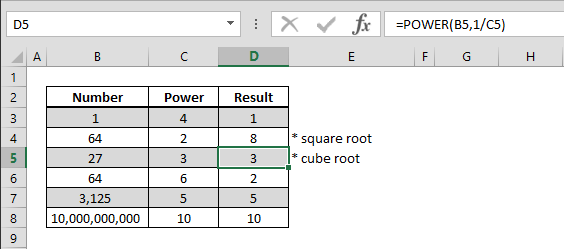## How to find the root of a number

Algebraically, the nth root of a number can be calculated by using 1/n for its power. You can always use caret symbol (^) to find a certain power of a number, but doing this with a formula can be easier to read and identify in nested formula sets. Syntax =POWER(base...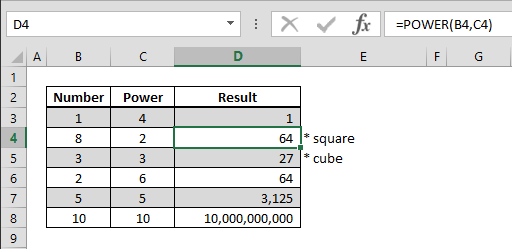## How to find the power of a number

You can always use caret symbol (^) to find a certain power of a number. However, doing this with a formula can be easier to read and identify in nested formula sets. Syntax =POWER(base number, how many times that base number will be multiplied by itself) Steps Begin...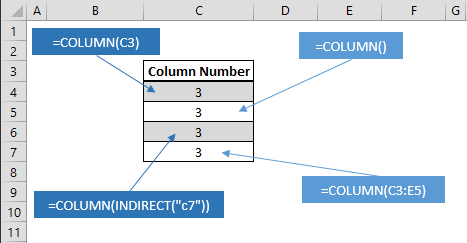## How to get the column index of a cell using the COLUMN function

Working with Excel’s coordinate system makes building data models extremely easy. You can retrieve the column index information of a cell using the COLUMN function and implement in other formulas. Syntax =COLUMN(cell or range reference you want get its column...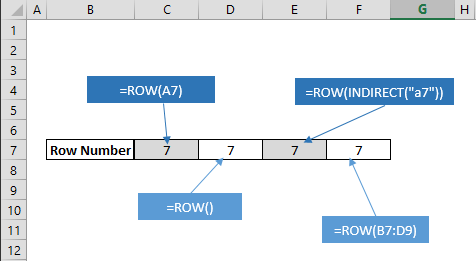## How to get the row index of a cell using the ROW function

Working with Excel’s coordinate system makes building data models extremely easy. You can retrieve the row index information of a cell using the ROW function and implement in other formulas. Syntax =ROW(cell or range reference you want get its row number) Steps...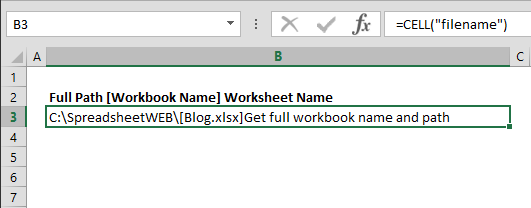## How to get the worksheet name, workbook name, and its path

Working across several workbooks with multiple sheets can be tedious. Furthermore, file names are dynamic and can be changed by your users. Use this simple trick to organize your data and label workbook details. Syntax =CELL(“filename”, reference which is...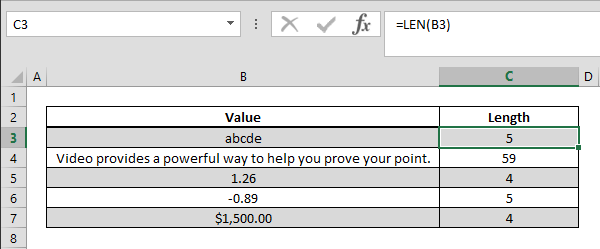## How to count the number of characters inside a cell

You can easily count the number of characters inside a cell using the LEN function. This function can be especially useful when identifying data types or creating validation rules. Syntax =LEN(text) Steps Begin by typing in =LEN( Select or type in the range reference...